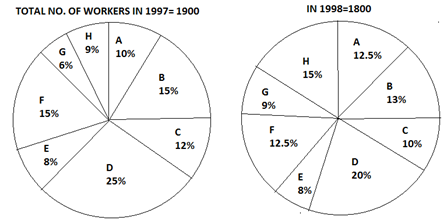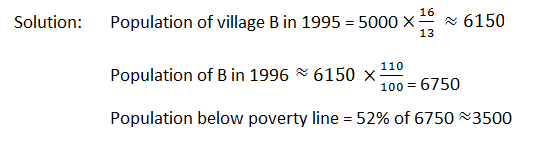New Students Offer - Use Code HELLO

# Data Interpretation - Double Diagram Pie Charts (2 Sets)

Data interpretation challenge for today :-

## Set 1

The following graph shows the no. of workers of different categories A, B, C, D, E, F, G and H of a factory for the two different years.Solution:

1997
1998
A
10% of 1900 = 190
12.5 % of 1800 = 225
B
285
234
C
228
180
D
475
360
E
152
144
F
285
225
G
114
162
H
171
270

#### Ques 1.

What is the total no. of increased workers for the categories in which the no. of workers has been increased?
Solution: The no. of workers has been increased in the category A (from 190 to 225 = 35), G (from 114 to 162 = 48) and H (from 171 to 270 = 99).
∴ Total no. of increased workers = 35 + 48 + 99 = 182.

#### Ques 2.

Find the percentage decrease in the no. of workers for the categories D and F taken together?
Solution: Reqd. percentage decrease#### Ques 3.

Which categories have shown the decrease in the no. of workers from 1997 to 1998?
Solution: Need to study the pie chart
Reqd. categories are B, C, D, E and F.

#### Ques 4.

Find the maximum possible difference of the no. of workers of any two categories taken together for one year and any two for the other year.
Solution: For the reqd. purpose, we have to select

(1) The two categories having the highest no of workers in 1997 and simultaneously the two categories having the least no. of workers in 1998 and
(2) The two categories having the highest no. of workers in 1998 and simultaneously the two categories having the least no. of workers in 1997.

#### Ques 5.

What is the difference between the no. of the category F for the two years and the same category for the two years?
Solution: The difference between the no. of workers of the category F for the two years = 285 – 225 = 60
And the percentage difference (15 – 12.5) = 2.5%
We have 100% = 3600
2.5 % = (360/100  ×2.5) = 90

## SET 2

Study the following chart to answer the questions:

Village % population below poverty line
A 45
B 52
C 38
D 58
E 46
F 49
G 51#### Ques 1.

In 1996, the population of villages A as well as B is increased by 10% from the year 1995. If the population of village A in 1995 was 5000 and the percentage of population below poverty line in 1996 remains same as in 1995, find approximately the population of village B below poverty line in 1996.#### Ques 2.

If in 1997 the population of village D is increased by 10% and the population of village G is reduced by 5% from 1995 and the population of village G in 1995 was 9000, what is the total population of villages D and G in 1997?
Solution: Population of village D in 1995 = 9000 ×17/15 = 10,200

Population of village D in 1997 = 10,200 ×110/100  = 11,220
Population of village G in 1997 = 9,000 ×95/100 = 8,550

Total population of villages D and G in 1997 = 11,220 + 8,550 = 19,770.

#### Ques 3.

If in 1995 the total population of the seven villages together was 55,000 approximately, what will be population of village F in that year below poverty line?
Solution: Population of village F below poverty line
= 55000 ×13/100 ×49/100  ≈3500

#### Ques 4.

If the population of village C below poverty line in 1995 was 1520, what was the population of village F in 1995?
Solution: Population of village F in 1995
= 1520 ×100/38 ×13/8=6500

#### Ques 5.

The population of village C is 2000 in 1995. What will be the ratio of population of village C below poverty line to that of the village E below poverty line in that year?